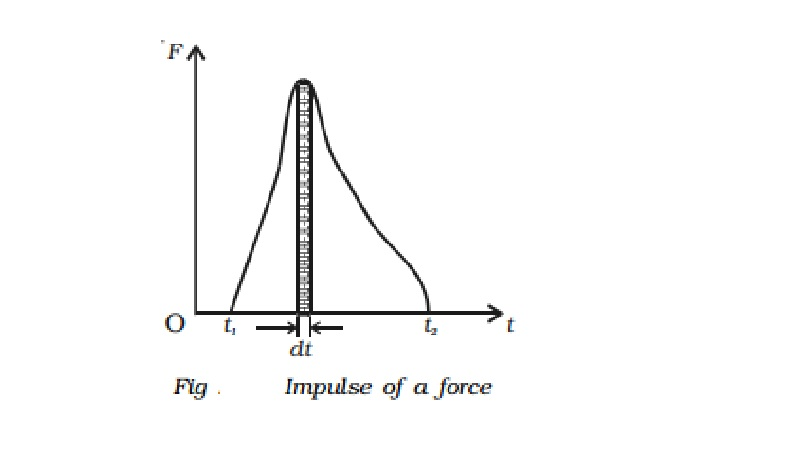Home | | Physics | | Physics | Impulsive force and Impulse of a force

# Impulsive force and Impulse of a forceAn impulsive force is a very great force acting for a very short time on a body, so that the change in the position of the body during the time the force acts on it may be neglected.

Impulsive force and Impulse of a force

(i) Impulsive Force

An impulsive force is a very great force acting for a very short time on a body, so that the change in the position of the body during the time the force acts on it may be neglected.

(e.g.) The blow of a hammer, the collision of two billiard balls etc.

(ii) Impulse of a force

The impulse J of a constant force F acting for a time t is defined as the product of the force and time.

(i.e) Impulse = Force x time

= F  x tThe impulse of force F acting over a time interval t is defined by the integral,The impulse of a force, therefore can be visualised as the area under the force versus time graph as shown in Fig.. When a variable force acting for a short interval of time, then the impulse can be measured as,

J = Faverage   dt              ....(2)

Impulse of a force is a vector quantity and its unit is N s.

Principle of impulse and momentum

By Newton's second law of motion, the force acting on a body = m a where m = mass of the body and a = acceleration produced

The impulse of the force = F x t = (m a) t

If u and be the initial and final velocities of the body then, a = (v-u)/ t

Therefore, impulse of the force = m [(v-u)/t ] t = m(v-u) = mv-mu

Impulse = final momentum of the body - initial momentum of the body.

Impulse of the force = Change in momentum

(i.e) Impulse of the force = Change in momentum

The above equation shows that the total change in the momentum of a body during a time interval is equal to the impulse of the force acting during the same interval of time. This is called principle of impulse and momentum.

Study Material, Lecturing Notes, Assignment, Reference, Wiki description explanation, brief detail

Related Topics